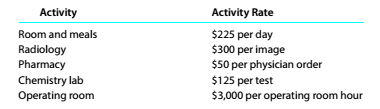Chapter 10, Problem 10.24E

Chapter
Section
Textbook Problem

Activity-based costing for a hospital Deer Lodge Regional Hospital plaits to use activity-based costing to assign hospital indirect costs to the care of patients. The hospital has identified the following activities and activity rates for the hospital indirect costs:The records of two representative patients were analyzed, using the activity rates. The activity information associated with the two patients is as follows:a. Determine the activity cost associated with each patient, b.Why is the total activity cost different for the two patients?

To determine

Concept Introduction:

Activity Based Costing: In this system of costing we attribute all the overhead cost as per the activity which is related to that cost or overhead. This system of costing also called ABC cost system. Many organizations consider this method of costing as the best method because this helps to attribute the cost to its respective cost centre.

Requirement-1:

To Calculate:

Determine the activity cost associates to each customer.

Explanation

Customer 1= Room and meals+ Radiology+ Pharmacy+ Chemistry lab+ Operating room

= 225*1+ 300*2+ 50*1+ 125*2+ 0.6*3000

= 225+ 600+ 50+ 250+ 1800

Custo...

To determine

Concept Introduction:

Activity Based Costing: In this system of costing we attribute all the overhead cost as per the activity which is related to that cost or overhead. This system of costing also called ABC cost system. Many organizations consider this method of costing as the best method because this helps to attribute the cost to its respective cost centre.

Requirement-2:

To Explain:

Why is the total activity cost different for the two patients?

Still sussing out bartleby?

Check out a sample textbook solution.

See a sample solution

The Solution to Your Study Problems

Bartleby provides explanations to thousands of textbook problems written by our experts, many with advanced degrees!

Get Started

Find more solutions based on key concepts